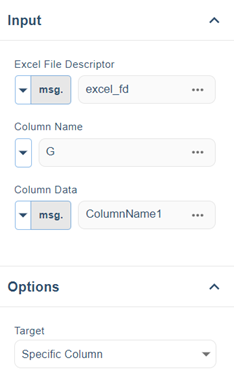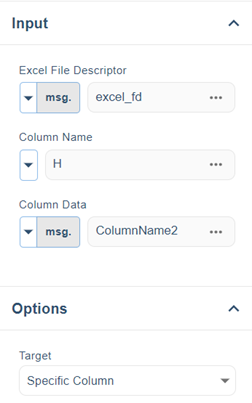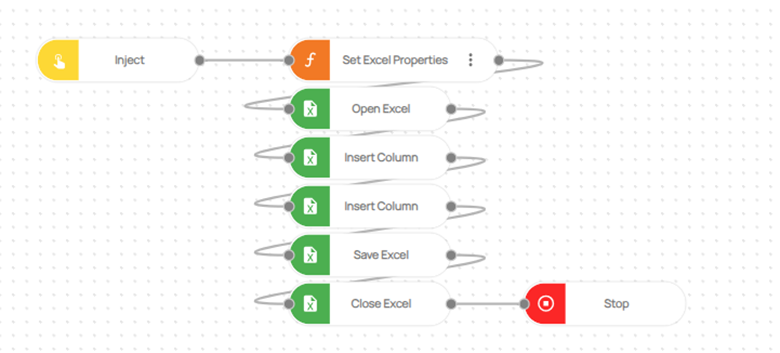# How to add two columns in Excel?

In the realm of data manipulation and analysis, Microsoft Excel stands as a cornerstone, offering a vast array of functions to run tasks and unearth insights from raw information. One of the fundamental operations that professionals frequently encounter is adding columns to Excel spreadsheets, a seemingly simple yet crucial task that can significantly enhance data organization and analysis.

In this blog post, we’ll dive focusing on the process of adding two columns within a spreadsheet.

To begin, Log in to your Robomotion workspace. If you haven't registered yet, you can create your workspace from the provided link. This flow utilizes Excel. If you attempt the same operations with the MS Excel Package, the flow will result in an error.

1. Use the 'Insert Column' node.
2. Begin by defining the column names.
3. Next, specify the particular column within the input of each 'Insert Column' node and add the desired data.
4. Lastly, employ the 'Save Excel' node to save the changes.

Flow Steps

1. The 'Inject' node is used to initialize the flow.
2. Three variables are defined using a "function" node. One of these variables is 'msg.excel_path', which specifies the path of the Excel file to be used. The other two variables are 'msg.ColumnName1' and 'msg.ColumnName2', both of which define column names

Code for Set Excel Properties Function Node:

``msg.excel_path = ""; // Give the excel path heremsg.ColumnName1 = [""]; // Give the column name heremsg.ColumnName2 = [""]; // Give the column name here/*Note!!Each insert column node is used to insert a column.The variables msg.ColumnData1 and msg.ColumnData2 are the column names to be added.*/return msg;``
1. 'Open Excel' node is used to open the defined Excel file. The input for the 'Open Excel' node is the variable 'msg.excel_path'.

2. The first column name that you've defined is included using the 'Insert Column' node. In this process, the 'msg.ColumnName1' variable serves as the input for the 'Insert Column' node, and this variable is then written into the Column Data field. Simultaneously, within the Column Name field, you specify the particular column where you wish to add this column name within the Excel file.1. The second column name that has been defined is added using the 'Insert Column' node. The input of the 'Insert Column' node is the variable 'msg.ColumnName2'. This variable is written into the Column Data field. Moreover, in the Column Name field, you define the specific column in which you want the column name to be included in the Excel file.1. The Excel file is saved at the designated Excel path. The 'Save Excel' node takes the variable 'msg.excel_path' as its input.
2. The Excel file that has been saved is closed by using the 'Close Excel' node
3. The 'Stop' node is used to stop the flow.Here you can access the complete flow by clicking on the following link: https://casestudies.robomotion.io/designer/shared/9Qiu4RfRmKiS1wiY7c5z95